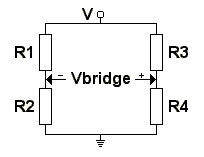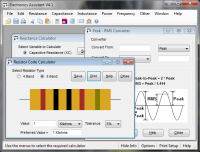# Wheatstone Bridge Calculator

The first of these calculators will calculate the fourth resistor value in a Wheatstone Bridge given the other three resistor values and the bridge being balanced. The second calculator will find the voltage across the bridge given the resistor values and supply voltage. Preferred resistor values are also calculated for the first calculator. Use the radio buttons on the calculator to select the preferred value series to use. More information about the Wheatstone Bridge can be found in the technical data section. The diagram below shows the notations used by the calculators:#### Calculate R4 for Balanced Bridge from R1 to R3:

 Enter R1: Ohms Kilohms Megohms Enter R2: Ohms Kilohms Megohms Enter R3: Ohms Kilohms Megohms Select preferred series (optional): E12 E24 E48 E96 E192 R4 = ? Preferred R4 = ?

#### Calculate Vbridge from R1 - R4 and Supply Voltage:

 Enter R1: Ohms Kilohms Megohms Enter R2: Ohms Kilohms Megohms Enter R3: Ohms Kilohms Megohms Enter R4: Ohms Kilohms Megohms Enter V: Volts Millivolts Microvolts Vbridge = ?

#### Formulae:

Bridge voltage, Vbridge:

Vbridge = ((R4 / (R3 + R4)) - (R2 / (R1 + R2))) * V

For a balanced bridge, the equivalent resistance between V and GND is:

RE = ((R1 + R2) * (R3 + R4)) / (R1 + R2 + R3 + R4)

See the technical data section for more details.

# If you found this calulator useful you should try Electronics Assistant!• All the online calculators and more in a stand-alone application
• Converts Resistor & Inductor colour codes, calculates LED series resistors, capacitance units, series / parallel resistors & capacitors, frequency, reactance & more
• Calculation of nearest preferred resistor values with a choice of 5 series from E12 to E192
• Print & save calculation results